### MEMO 2014 ekipno problem 5

Kvaliteta:
Avg: 3.0
Težina:
Avg: 5.5
Let$ABC$ be a triangle with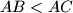$AB < AC$. Its incircle with centre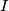$I$ touches the sides$BC$,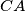$CA$, and$AB$ in the points$D$,$E$, and$F$ respectively. The angle bisector$AI$ intersects the lines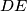$DE$ and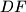$DF$ in the points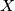$X$ and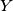$Y$ respectively. Let$Z$ be the foot of the altitude through$A$ with respect to$BC$.

Prove that$D$ is the incentre of the triangle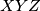$XYZ$.
Source: Srednjoeuropska matematička olimpijada 2014, ekipno natjecanje, problem 5